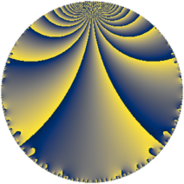# Properties

 Label 43.5.fLevel $43$ Weight $5$ Character orbit 43.f Rep. character $\chi_{43}(2,\cdot)$ Character field $\Q(\zeta_{14})$ Dimension $78$ Newform subspaces $1$ Sturm bound $18$ Trace bound $0$

# Related objects

## Defining parameters

 Level: $$N$$ $$=$$ $$43$$ Weight: $$k$$ $$=$$ $$5$$ Character orbit: $$[\chi]$$ $$=$$ 43.f (of order $$14$$ and degree $$6$$) Character conductor: $$\operatorname{cond}(\chi)$$ $$=$$ $$43$$ Character field: $$\Q(\zeta_{14})$$ Newform subspaces: $$1$$ Sturm bound: $$18$$ Trace bound: $$0$$

## Dimensions

The following table gives the dimensions of various subspaces of $$M_{5}(43, [\chi])$$.

Total New Old
Modular forms 90 90 0
Cusp forms 78 78 0
Eisenstein series 12 12 0

## Trace form

 $$78q - 7q^{2} - 7q^{3} + 69q^{4} - 7q^{5} - 140q^{6} - 343q^{8} + 486q^{9} + O(q^{10})$$ $$78q - 7q^{2} - 7q^{3} + 69q^{4} - 7q^{5} - 140q^{6} - 343q^{8} + 486q^{9} - 189q^{10} - 26q^{11} - 119q^{12} + 608q^{13} + 45q^{14} - 797q^{15} + 593q^{16} - 188q^{17} - 574q^{18} + 1421q^{19} - 2107q^{20} + 2952q^{21} - 2359q^{22} + 652q^{23} - 253q^{24} + 1264q^{25} - 2023q^{26} - 7q^{27} + 1785q^{28} - 2317q^{29} - 252q^{30} + 5714q^{31} - 6517q^{32} + 9023q^{33} - 679q^{34} + 1374q^{35} - 8544q^{36} + 95q^{38} - 10339q^{39} - 5901q^{40} - 3611q^{41} + 7001q^{43} + 8148q^{44} + 8862q^{45} - 2660q^{46} + 6268q^{47} + 11081q^{48} - 1074q^{49} - 17353q^{51} + 8277q^{52} - 200q^{53} - 8147q^{54} + 9863q^{55} + 15927q^{56} - 25075q^{57} + 13045q^{58} + 1468q^{59} - 357q^{60} - 8239q^{61} + 10073q^{62} - 875q^{63} + 1173q^{64} - 19159q^{65} - 24551q^{66} + 42658q^{67} + 3910q^{68} - 903q^{69} + 37870q^{70} + 8960q^{71} - 40586q^{72} + 13342q^{73} + 37116q^{74} - 44996q^{75} - 60438q^{76} + 2625q^{77} - 13562q^{78} - 48318q^{79} + 1048q^{81} - 57211q^{82} + 17014q^{83} - 50382q^{84} + 21461q^{86} - 6174q^{87} + 34909q^{88} + 51590q^{89} - 6494q^{90} + 35840q^{91} + 105420q^{92} - 38115q^{94} + 65915q^{95} + 101606q^{96} - 18427q^{97} + 63455q^{98} + 36377q^{99} + O(q^{100})$$

## Decomposition of $$S_{5}^{\mathrm{new}}(43, [\chi])$$ into newform subspaces

Label Dim. $$A$$ Field CM Traces $q$-expansion
$$a_2$$ $$a_3$$ $$a_5$$ $$a_7$$
43.5.f.a $$78$$ $$4.445$$ None $$-7$$ $$-7$$ $$-7$$ $$0$$